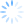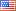Measure what you can measure, I believe in order to understand the problem of productivity is by measuring the velocity of the developer based on the workload. The question is how to measure the developer workload. let's discuss on this article.

# The Metrics

the metric that can be measured in various way. In Agile, we will use story point. However, story point is relative measurement. 1 point in X team will be different in 1 point in Y Team. Therefore, the metric is relative and can be converted through several ways.

Let's take example one of user story in e-commerce system.

"user want to register to the web so that they can start to sell."

the three developers will be developed the user story. One developer is programmer, one as database engineer, and the last one is user interface designer.

each of the developer gives the estimation points.

1. Programmer - 8
2. Database Engineer - 5
3. User Interface - 5

They can use the agreement between members or doing vote till they have similar values. At the end of the discussion, they agree to give 8 points.

# The reality

What is the meaning of 8 points. is it mean 8 days? 8 hours? how we can convert the points into others meaningful value such as time, budget, or even line of codes.

Let's take one approach, we want to convert into a man-hour. In order to do that, you need to find time (t)

if the story points is symbolized s. Based on physics we can find the t by dividing the story point (s) with the developer velocity (v) simply states

t = s / v

However, we only have value for s, we don't have v! In order to understand the velocity, you can do the technique.

namely. historical analysis from the previous project.

Let's take example.

The 'similar' project in creating e-commerce can be finished in 1250 hours. it has with 250 story points. We can find the velocity by dividing s with t so we have

velocity = 250 / 1250

v = 0.2 points / hour

Based on that case, in the previous user story we will have

t = 8 / 0.2 = 40 hours

However, the 40 hours are good estimated. Based on Boehm the cone of uncertainty you will have between = 160 hours (worst case) and 10 hours for the good case (4x or 1/4 times)

So based on that assumption, we can propose that for developer that work for 100 points they will work for

T = s / v

Or

100 / 0.2 = 500 hours

If the project is 300 story points, it will take about 1500 hours.

If the project will have three developers and all developers work with same full-time commitment it means. The developers will work for 40 hours / week (assumption five days of work and 8 hours/day) or 120 hours for total or 480 hours / month.

Based on the estimation the project will run for

1500 / 480 = 3.125 Months

In the reality, each developer will be assigned with the different task so that they will have different workload.

# The money.

Now you can convert the project based on the story points. For example, the budget of the project is 1800 USD and has 300 story points. It means each point will have 1800 / 300 = 6 USD / point.

In the end of the month. For the developer that can finish 100 points will obtain 600 USD• Comment
• Preview#### Get in touch